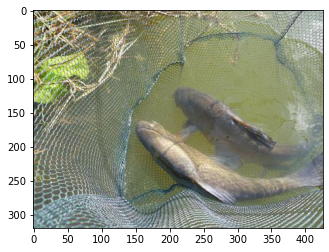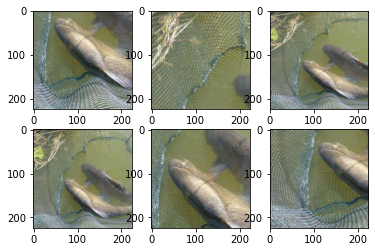Crop the given PIL Image to random size and aspect ratio with random interpolation.

In this piece of documentation, we will be looking at the RandomResizedCropAndInterpolation data augmentation in timm. This augmentation get's applied in timm to the input data by default unless the --no-aug flag has been passed to train the model, in which case no augmentations except Resize and CenterCrop get applied.

Since this RandomResizedCropAndInterpolation augmentation get's applied by default, we don't look into an example on how we could apply it to the training data. Any training script applies this technique such as the one below:

python train.py ../imagenette2-320


To not apply any data augmentation to the input data, one could pass in the --no-aug flag like so:

python train.py ../imagenette2-320 --no-aug


## RandomResizedCropAndInterpolation as a standalone data augmentation technique for custom training loop

In this section we will be looking at how we could leverage the timm library to apply this data augmentation technique to our input data. Let's see an example.

from timm.data.transforms import RandomResizedCropAndInterpolation
from PIL import Image
from matplotlib import pyplot as plt

tfm = RandomResizedCropAndInterpolation(size=224)
X   = Image.open("../../imagenette2-320/train/n01440764/ILSVRC2012_val_00000293.JPEG")
plt.imshow(X)

<matplotlib.image.AxesImage at 0x7f8788f027f0>As usual, we create an input image X which is the usual image of a "tench" as used everywhere else in this documentation.

Let's now apply the transform multiple times and visualize the results.

for i in range(6):
plt.subplot(2, 3, i+1)
plt.imshow(tfm(X))As can be seen below, we can see the transform is working and it is randomly cropping/resizing the input image and also randomly changing the aspect ratio of the image.